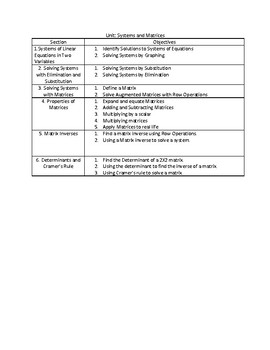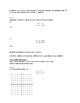# Algebra 2: Systems and Matrices NotesSubject
Grade Levels
Resource Type
Common Core Standards
Product Rating
File Type

PDF (Acrobat) Document File

Be sure that you have an application to open this file type before downloading and/or purchasing.

691 KB|24 pages
Share
Product Description

A detailed guided notes packet which includes a paragraph introduction to the topic, a worked out example of the procedureal task and many excerise examples that you can do with your students in class.

Content:

Section 1: Introduction to Systems as common solutions to graphs, Solve two-equation linear systems using graphing, inconsistent systems of parallel lines and identical lines.

Section 2: Solving two-equation linear systems using substitution and elimination, Applications of two-equation linear systems, brief introduction to a three-equation system and solving using elimination.

Section 3: Introduction to matrices, operations on Matrices (addition, subtraction, scalar and matrix multiplication,) Applied problem with matrice operations.

Section 4: Defining the indentiy matrix, Solving two-equation linear systems with matrices using row operations, Inconsistent systems in a matrix. Brief dicussion of three-equation system solved with row operations.

Section 5: Introduction of the determinant, finding the inverse of a matrix by using the determinant. (note: finding the inverse using row operations is not covered.) Solving two-equation linear systems with matrices inverses and matrix multiplication. Inconstistent system example.

Section 6: Solving two-equation linear systems with matrices using Cramer's Rule. Proof of Cramer's rule explained. Inconsitent system example. Applications of a system using any method for solving.

Total Pages
24 pages
Answer Key
Not Included
Teaching Duration
N/A
Report this ResourceSign Up